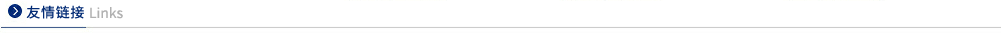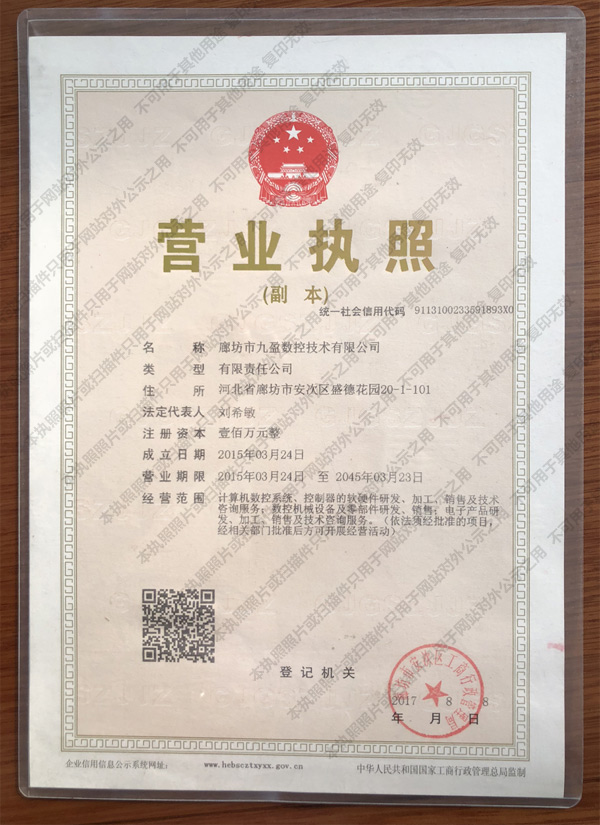Axis Count in Motion Controllers

Motion controllers are an essential component of many automated systems, providing precise control over the movement of motors, actuators, and other components. The number of axes in a motion controller determines the number of degrees of freedom that can be controlled, and is an important factor in determining the overall performance of the system.

Axis count is typically expressed as a number of axes, such as 3, 4, or 6. The number of axes in a motion controller is determined by the number of motors or actuators that need to be controlled. For example, a 3-axis motion controller would be used to control three motors or actuators, while a 6-axis motion controller would be used to control six motors or actuators.

The number of axes in a motion controller also affects the type of motion that can be achieved. For example, a 3-axis motion controller can provide linear motion along three axes, while a 6-axis motion controller can provide linear motion along six axes, as well as rotational motion.

The number of axes in a motion controller also affects the accuracy of the motion. Generally, the more axes a motion controller has, the more accurate the motion will be. This is because each axis can be controlled independently, allowing for more precise control over the motion.

In addition to the number of axes, other factors such as the type of motors or actuators used, the type of motion control algorithm, and the type of feedback system can also affect the performance of a motion controller.

Overall, the number of axes in a motion controller is an important factor in determining the performance of the system. By understanding the number of axes and the type of motion that can be achieved, engineers can select the best motion controller for their application.